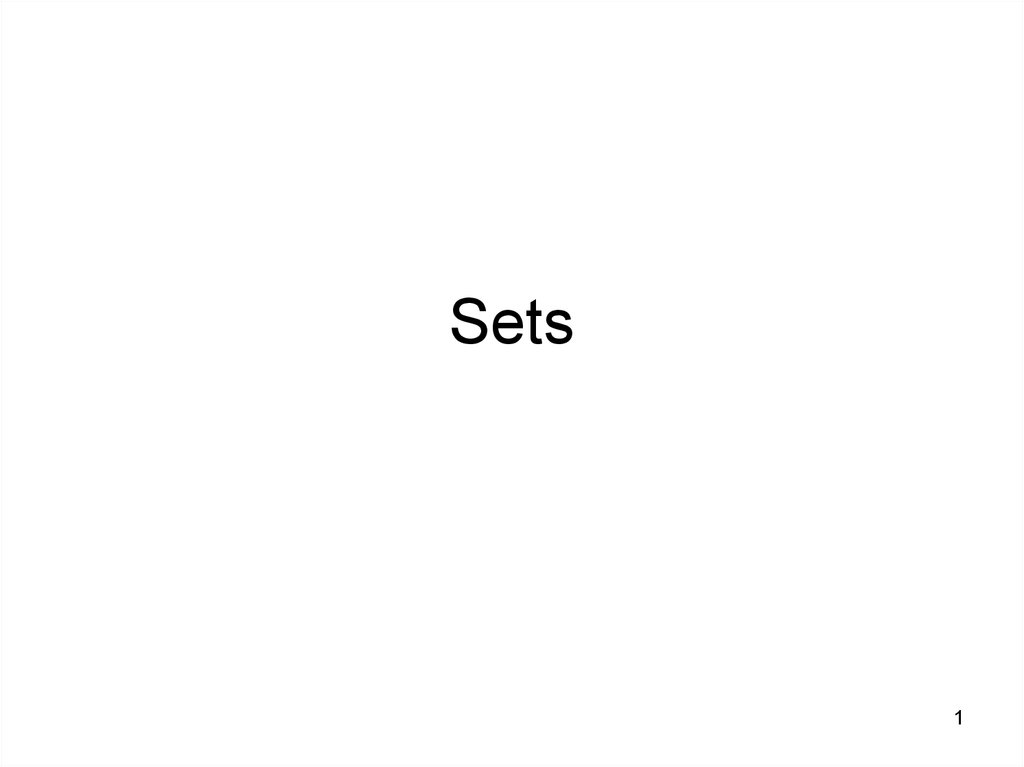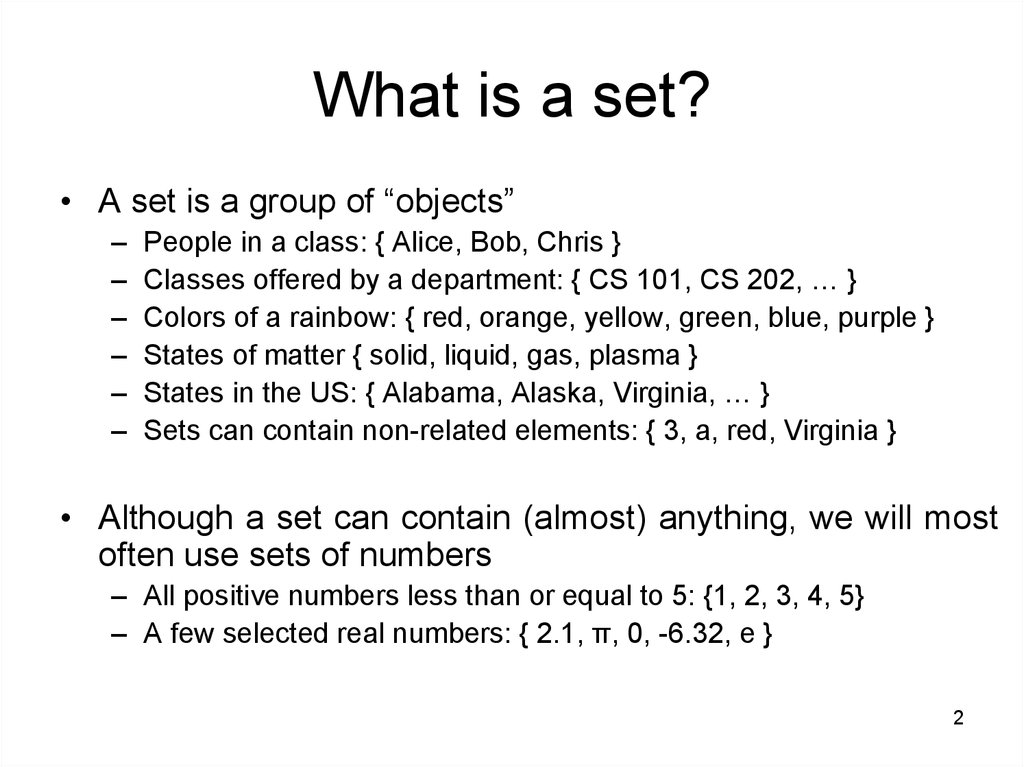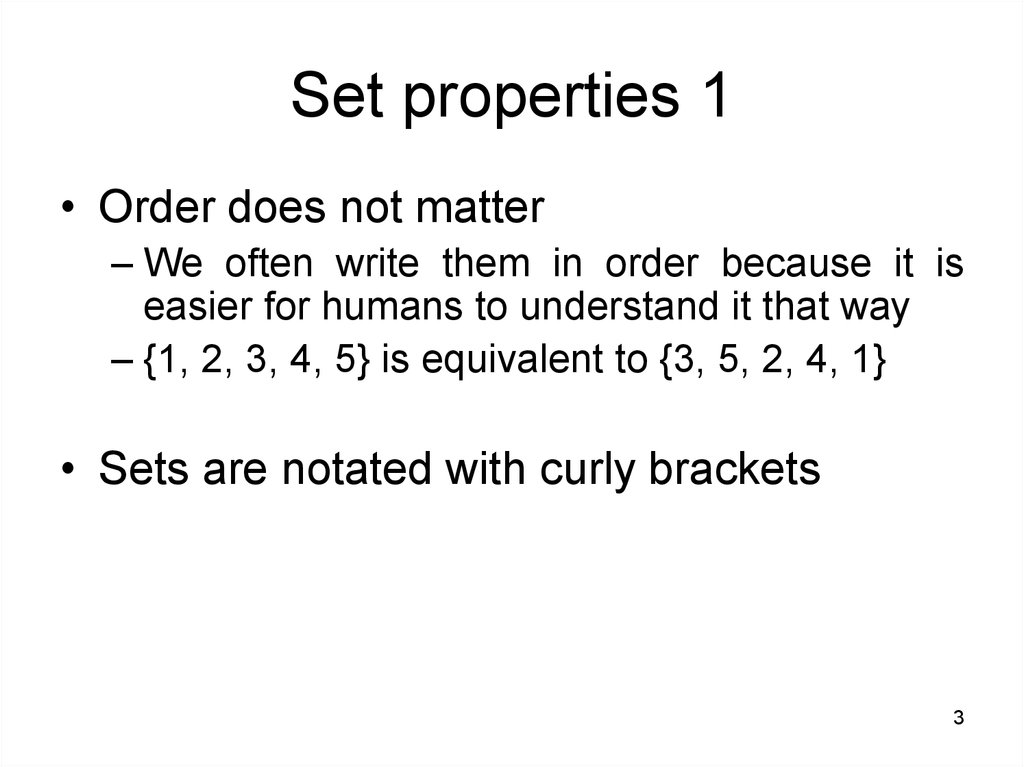# Discrete mathematics. Sets

1

## 2. What is a set?

• A set is a group of “objects”

People in a class: { Alice, Bob, Chris }
Classes offered by a department: { CS 101, CS 202, … }
Colors of a rainbow: { red, orange, yellow, green, blue, purple }
States of matter { solid, liquid, gas, plasma }
States in the US: { Alabama, Alaska, Virginia, … }
Sets can contain non-related elements: { 3, a, red, Virginia }
• Although a set can contain (almost) anything, we will most
often use sets of numbers
– All positive numbers less than or equal to 5: {1, 2, 3, 4, 5}
– A few selected real numbers: { 2.1, π, 0, -6.32, e }
2

## 3. Set properties 1

• Order does not matter
– We often write them in order because it is
easier for humans to understand it that way
– {1, 2, 3, 4, 5} is equivalent to {3, 5, 2, 4, 1}
• Sets are notated with curly brackets
3

## 4. Set properties 2

• Sets do not have duplicate elements
– Consider the set of vowels in the alphabet.
• It makes no sense to list them as {a, a, a, e, i, o, o,
o, o, o, u}
• What we really want is just {a, e, i, o, u}
– Consider the list of students in this class
• Again, it does not make sense to list somebody
twice
• Note that a list is like a set, but order does
matter and duplicate elements are allowed
– We won’t be studying lists much in this class
4

## 5. Specifying a set 1

• Sets are usually represented by a capital
letter (A, B, S, etc.)
• Elements are usually represented by an
italic lower-case letter (a, x, y, etc.)
• Easiest way to specify a set is to list all the
elements: A = {1, 2, 3, 4, 5}
– Not always feasible for large or infinite sets
5

## 6. Specifying a set 2

• Can use an ellipsis (…): B = {0, 1, 2, 3, …}
– Can cause confusion. Consider the set C = {3, 5, 7,
…}. What comes next?
– If the set is all odd integers greater than 2, it is 9
– If the set is all prime numbers greater than 2, it is 11
• Can use set-builder notation

D = {x | x is prime and x > 2}
E = {x | x is odd and x > 2}
The vertical bar means “such that”
Thus, set D is read (in English) as: “all elements x
such that x is prime and x is greater than 2”
6

## 7. Specifying a set 3

• A set is said to “contain” the various
“members” or “elements” that make up the
set
– If an element a is a member of (or an element
of) a set S, we use then notation a S
• 4 {1, 2, 3, 4}
– If an element is not a member of (or an
element of) a set S, we use the notation a S
• 7 {1, 2, 3, 4}
• Virginia {1, 2, 3, 4}
7

## 8. Often used sets

• N = {0, 1, 2, 3, …} is the set of natural numbers
• Z = {…, -2, -1, 0, 1, 2, …} is the set of integers
• Z+ = {1, 2, 3, …} is the set of positive integers
(a.k.a whole numbers)
– Note that people disagree on the exact definitions of
whole numbers and natural numbers
• Q = {p/q | p Z, q Z, q ≠ 0} is the set of
rational numbers
– Any number that can be expressed as a fraction of
two integers (where the bottom one is not zero)
• R is the set of real numbers
8

## 9. The universal set 1

• U is the universal set – the set of all of
elements (or the “universe”) from which
given any set is drawn
– For the set {-2, 0.4, 2}, U would be the real
numbers
– For the set {0, 1, 2}, U could be the natural
numbers (zero and up), the integers, the
rational numbers, or the real numbers,
depending on the context
9

## 10. The universal set 2

– For the set of the students in this class, U
would be all the students in the University (or
perhaps all the people in the world)
– For the set of the vowels of the alphabet, U
would be all the letters of the alphabet
– To differentiate U from U (which is a set
operation), the universal set is written in a
different font (and in bold and italics)
10

## 11. Venn diagrams

• Represents sets graphically
– The box represents the universal set
– Circles represent the set(s)
• Consider set S, which is
the set of all vowels in the
alphabet
• The individual elements
are usually not written
in a Venn diagram
b
c
d f
g
h
k
l
j
m
n
p
q
r
s
t
v
w
x
y
z
U
S
e
a
o
i
u
11

## 12. Sets of sets

• Sets can contain other sets
– S = { {1}, {2}, {3} }
– T = { {1}, {{2}}, {{{3}}} }
– V = { {{1}, {{2}}}, {{{3}}}, { {1}, {{2}}, {{{3}}} } }
• V has only 3 elements!
• Note that 1 ≠ {1} ≠ {{1}} ≠ {{{1}}}
– They are all different
12

## 13. The empty set 1

• If a set has zero elements, it is called the
empty (or null) set
– Written using the symbol
– Thus, = { }
VERY IMPORTANT
– If you get confused about the empty set in a
problem, try replacing by { }
• As the empty set is a set, it can be a
element of other sets
– { , 1, 2, 3, x } is a valid set
13

## 14. The empty set 1

• Note that ≠ { }
– The first is a set of zero elements
– The second is a set of 1 element (that one
element being the empty set)
• Replace by { }, and you get: { } ≠ { { } }
• It’s easier to see that they are not equal that way
14

## 15. Set equality

• Two sets are equal if they have the same
elements
– {1, 2, 3, 4, 5} = {5, 4, 3, 2, 1}
• Remember that order does not matter!
– {1, 2, 3, 2, 4, 3, 2, 1} = {4, 3, 2, 1}
• Remember that duplicate elements do not matter!
• Two sets are not equal if they do not have
the same elements
– {1, 2, 3, 4, 5} ≠ {1, 2, 3, 4}
15

## 16. Subsets 1

• If all the elements of a set S are also elements of
a set T, then S is a subset of T
– For example, if S = {2, 4, 6} and T = {1, 2, 3, 4, 5, 6,
7}, then S is a subset of T
– This is specified by S T
• Or by {2, 4, 6} {1, 2, 3, 4, 5, 6, 7}
• If S is not a subset of T, it is written as such:
S T
– For example, {1, 2, 8} {1, 2, 3, 4, 5, 6, 7}
16

## 17. Subsets 2

• Note that any set is a subset of itself!
– Given set S = {2, 4, 6}, since all the elements
of S are elements of S, S is a subset of itself
– This is kind of like saying 5 is less than or
equal to 5
– Thus, for any set S, S S
17

## 18. Subsets 3

• The empty set is a subset of all sets (including
itself!)
– Recall that all sets are subsets of themselves
• All sets are subsets of the universal set
• A horrible way to define a subset:
– x ( x A x B )
– English translation: for all possible values of x,
(meaning for all possible elements of a set), if x is an
element of A, then x is an element of B
– This type of notation will be gone over later
18

## 19. Proper Subsets 1

• If S is a subset of T, and S is not equal to
T, then S is a proper subset of T
– Let T = {0, 1, 2, 3, 4, 5}
– If S = {1, 2, 3}, S is not equal to T, and S is a
subset of T
– A proper subset is written as S T
– Let R = {0, 1, 2, 3, 4, 5}. R is equal to T, and
thus is a subset (but not a proper subset) or T
• Can be written as: R T and R T (or just R = T)
– Let Q = {4, 5, 6}. Q is neither a subset or T
nor a proper subset of T
19

## 20. Proper Subsets 2

• The difference between “subset” and
“proper subset” is like the difference
between “less than or equal to” and “less
than” for numbers
• The empty set is a proper subset of all
sets other than the empty set (as it is
equal to the empty set)
20

S R
U
R
S
21

## 22. Set cardinality

• The cardinality of a set is the number of
elements in a set
– Written as |A|
• Examples
– Let R = {1, 2, 3, 4, 5}. Then |R| = 5
– | | = 0
– Let S = { , {a}, {b}, {a, b}}. Then |S| = 4
• This is the same notation used for vector length
in geometry
• A set with one element is sometimes called a
22
singleton set

## 23. Power sets 1

• Given the set S = {0, 1}. What are all the
possible subsets of S?
– They are: (as it is a subset of all sets), {0},
{1}, and {0, 1}
– The power set of S (written as P(S)) is the set
of all the subsets of S
– P(S) = { , {0}, {1}, {0,1} }
• Note that |S| = 2 and |P(S)| = 4
23

## 24. Power sets 2

• Let T = {0, 1, 2}. The P(T) = { , {0}, {1},
{2}, {0,1}, {0,2}, {1,2}, {0,1,2} }
• Note that |T| = 3 and |P(T)| = 8
• P( ) = { }
• Note that | | = 0 and |P( )| = 1
• If a set has n elements, then the power set
will have 2n elements
24

## 25. Tuples

• In 2-dimensional space, it is a (x, y) pair of
numbers to specify a location
• In 3-dimensional (1,2,3) is not the same as
(3,2,1) – space, it is a (x, y, z) triple of numbers
+y
• In n-dimensional space, it is a
n-tuple of numbers
– Two-dimensional space uses
pairs, or 2-tuples
– Three-dimensional space uses
triples, or 3-tuples
(2,3)
+x
• Note that these tuples are
ordered, unlike sets
– the x value has to come first
25

## 26. Cartesian products 1

• A Cartesian product is a set of all ordered 2tuples where each “part” is from a given set
– Denoted by A x B, and uses parenthesis (not curly
brackets)
– For example, 2-D Cartesian coordinates are the set of
all ordered pairs Z x Z
• Recall Z is the set of all integers
• This is all the possible coordinates in 2-D space
– Example: Given A = { a, b } and B = { 0, 1 }, what is
their Cartiesian product?
• C = A x B = { (a,0), (a,1), (b,0), (b,1) }
26

## 27. Cartesian products 2

• Note that Cartesian products have only 2
parts in these examples (later examples
have more parts)
• Formal definition of a Cartesian product:
– A x B = { (a,b) | a A and b B }
27

## 28. Cartesian products 3

• All the possible grades in this class will be a
Cartesian product of the set S of all the students
in this class and the set G of all possible grades
– Let S = { Alice, Bob, Chris } and G = { A, B, C }
– D = { (Alice, A), (Alice, B), (Alice, C), (Bob, A), (Bob,
B), (Bob, C), (Chris, A), (Chris, B), (Chris, C) }
– The final grades will be a subset of this: { (Alice, C),
(Bob, B), (Chris, A) }
• Such a subset of a Cartesian product is called a relation
(more on this later in the course)
28

## 29. Cartesian products 4

• There can be Cartesian products on more
than two sets
• A 3-D coordinate is an element from the
Cartesian product of Z x Z x Z
29

30

AUB
U
A
B
31

## 32. Set operations: Union

• Formal definition for the union of two sets:
A U B = { x | x A or x B }
• Further examples
– {1, 2, 3} U {3, 4, 5} = {1, 2, 3, 4, 5}
– {New York, Washington} U {3, 4} = {New
York, Washington, 3, 4}
– {1, 2} U = {1, 2}
32

## 33. Set operations: Union

• Properties of the union operation
–AU =A
–AUU=U
–AUA=A
–AUB=BUA
– A U (B U C) = (A U B) U C
Identity law
Domination law
Idempotent law
Commutative law
Associative law
33

A∩B
U
A
B
34

## 35. Set operations: Intersection

• Formal definition for the intersection of two
sets: A ∩ B = { x | x A and x B }
• Further examples
– {1, 2, 3} ∩ {3, 4, 5} = {3}
– {New York, Washington} ∩ {3, 4} =
• No elements in common
– {1, 2} ∩ =
• Any set intersection with the empty set yields the
empty set
35

## 36. Set operations: Intersection

• Properties of the intersection operation
–A∩U=A
–A∩ =
–A∩A=A
–A∩B=B∩A
– A ∩ (B ∩ C) = (A ∩ B) ∩ C
Identity law
Domination law
Idempotent law
Commutative law
Associative law
36

## 37. Disjoint sets 1

• Two sets are disjoint if the
have NO elements in
common
• Formally, two sets are
disjoint if their intersection
is the empty set
• Another example:
the set of the even
numbers and the
set of the odd
numbers
37

U
A
B
38

## 39. Disjoint sets 3

• Formal definition for disjoint sets: two sets
are disjoint if their intersection is the empty
set
• Further examples
– {1, 2, 3} and {3, 4, 5} are not disjoint
– {New York, Washington} and {3, 4} are
disjoint
– {1, 2} and are disjoint
• Their intersection is the empty set
– and are disjoint!
• Their intersection is the empty set
39

A-B
U
A
B
40

## 41. Set operations: Difference

• Formal definition for the difference of two
sets:
A - B = { x | x_ A and x B }
A - B = A ∩ B Important!
• Further examples
– {1, 2, 3} - {3, 4, 5} = {1, 2}
– {New York, Washington} - {3, 4} = {New York,
Washington}
– {1, 2} - = {1, 2}
• The difference of any set S with the empty set will
41
be the set S

## 42. Set operations: Symmetric Difference

• A symmetric difference of the sets contains all
the elements in either set but NOT both
• Formal definition for the symmetric difference of
two sets:
A B = { x | (x A or x B) and x A ∩ B}
A B = (A U B) – (A ∩ B) Important!
• Further examples
– {1, 2, 3} {3, 4, 5} = {1, 2, 4, 5}
– {New York, Washington} {3, 4} = {New York,
Washington, 3, 4}
– {1, 2} = {1, 2}
42
• The symmetric difference of any set S with the empty set will
be the set S

## 43. Complement sets

• A complement of a set is all the elements
that are NOT in the set
• Formal definition for the complement of a
set: A = { x | x A }
• Further examples (assuming U = Z)
– {1, 2, 3} = { …, -2, -1, 0, 4, 5, 6, … }
43

## 44. Complement sets

• Properties of complement sets
¯
¯
–A=A
¯
–AUA
=U
¯ =
–A∩A
Complementation law
Complement law
Complement law
44